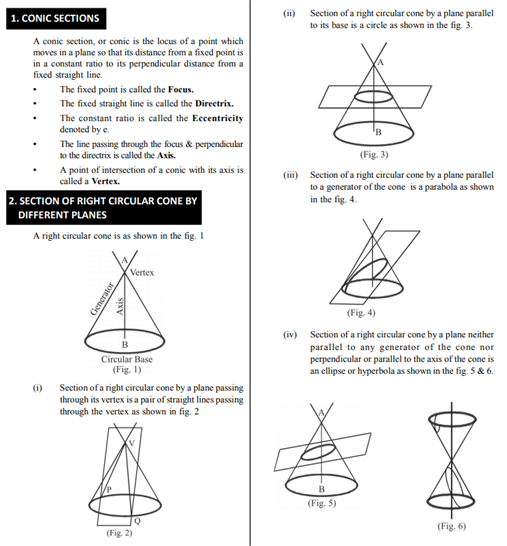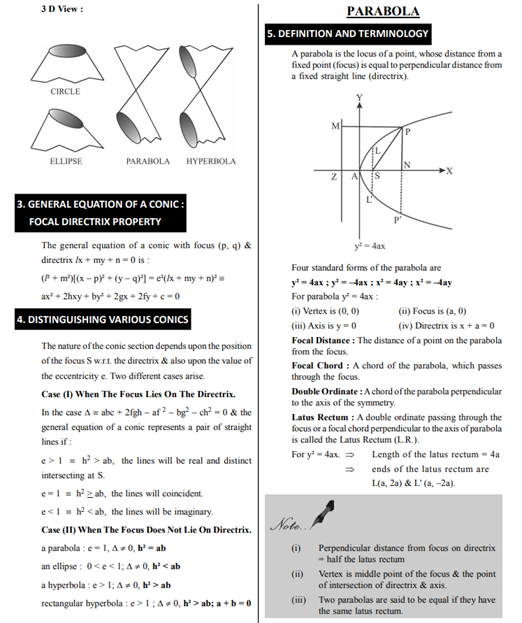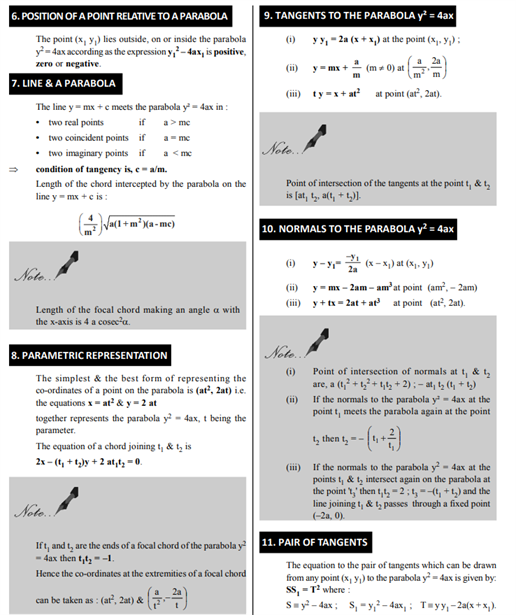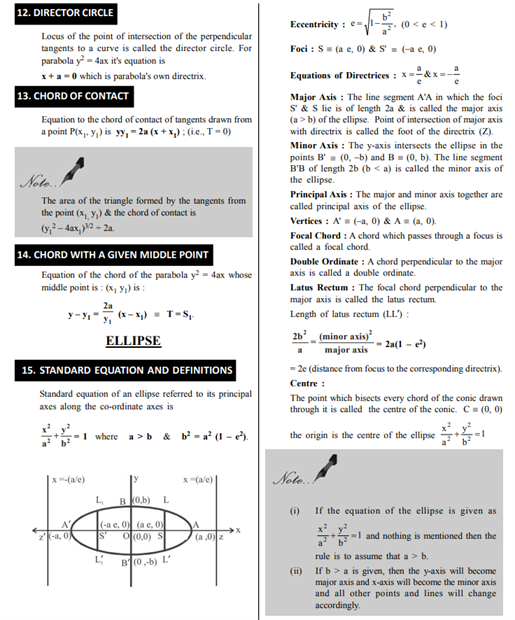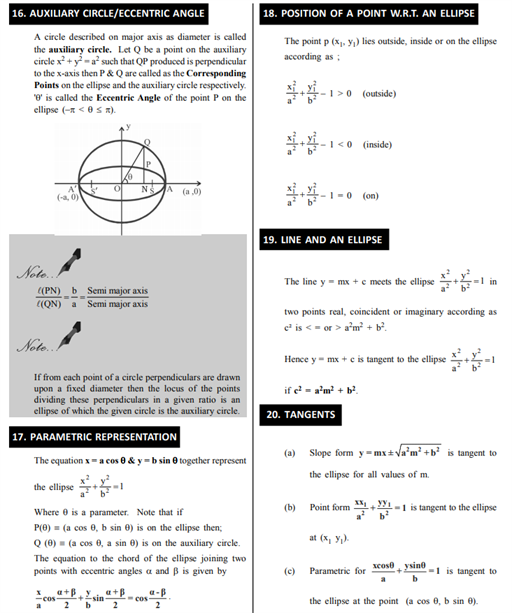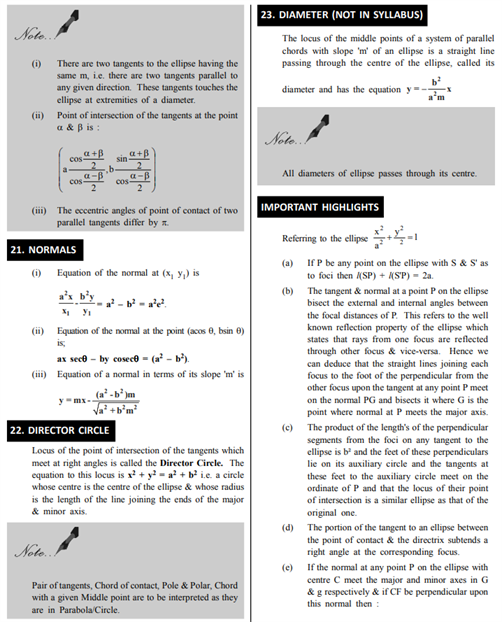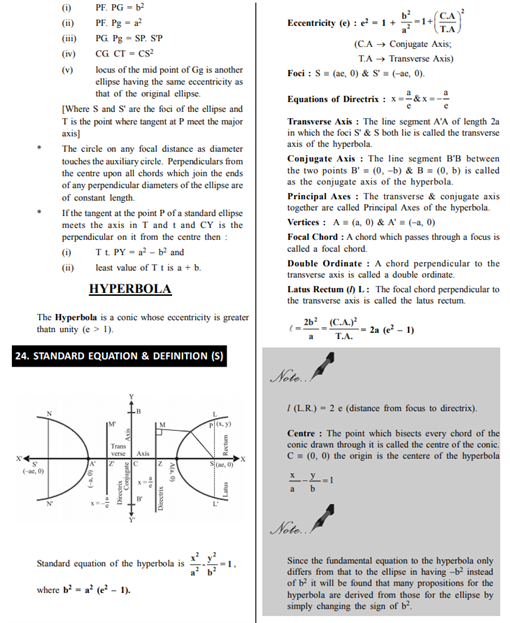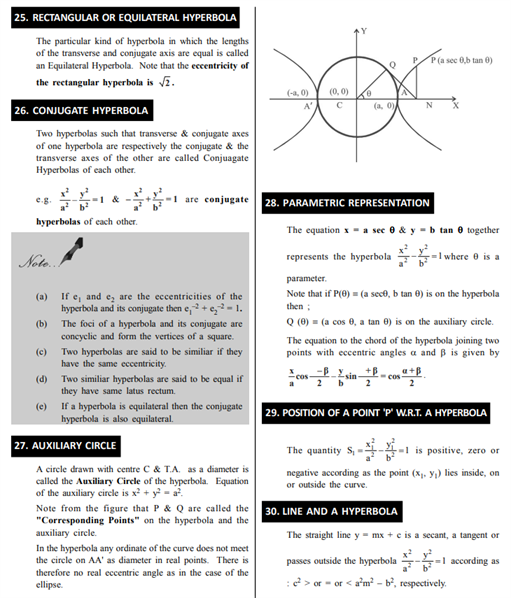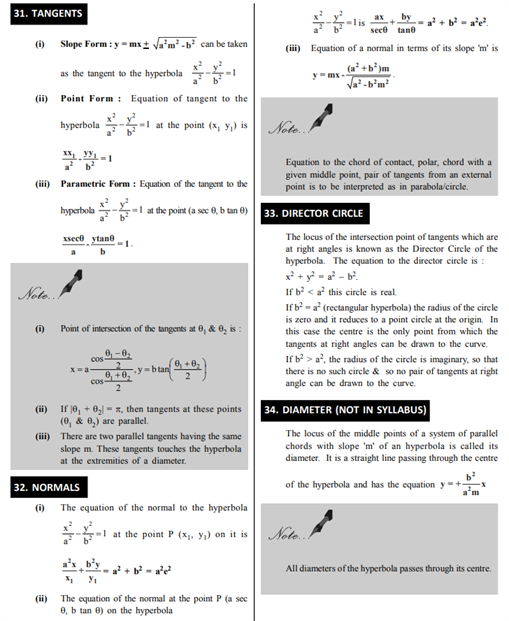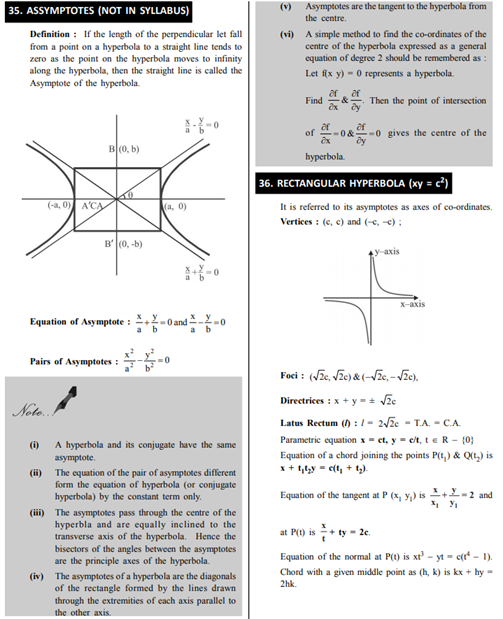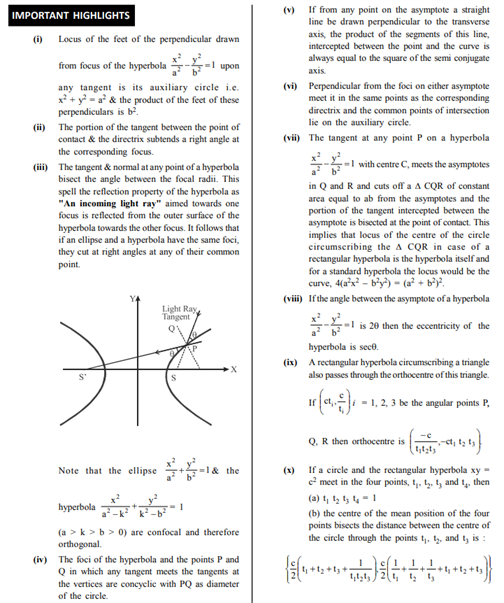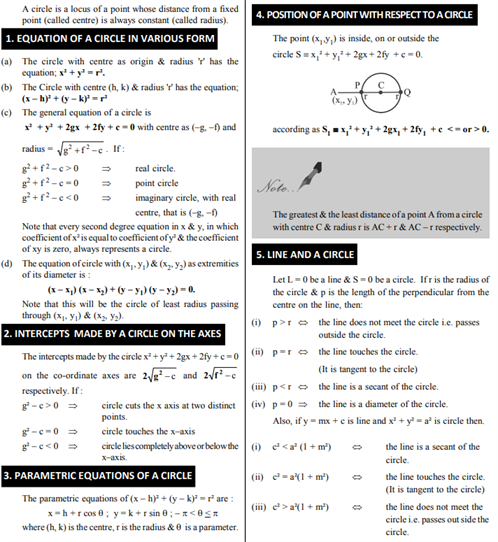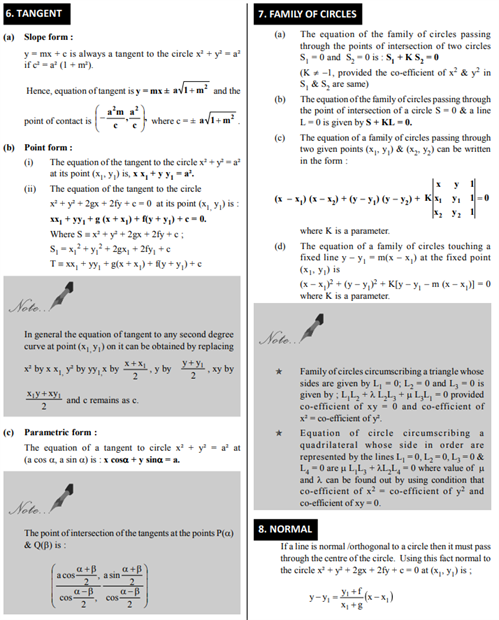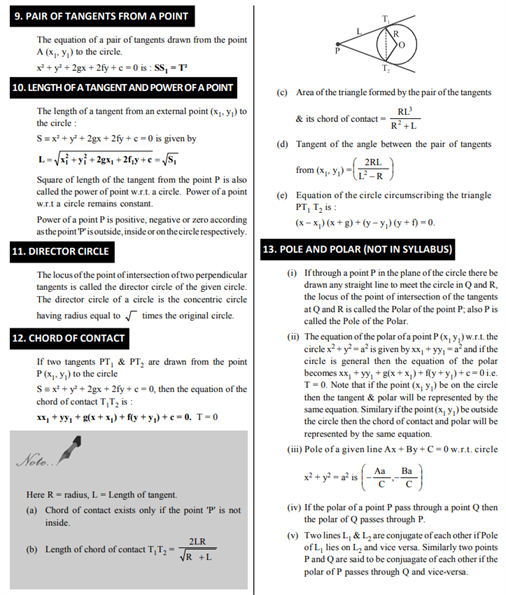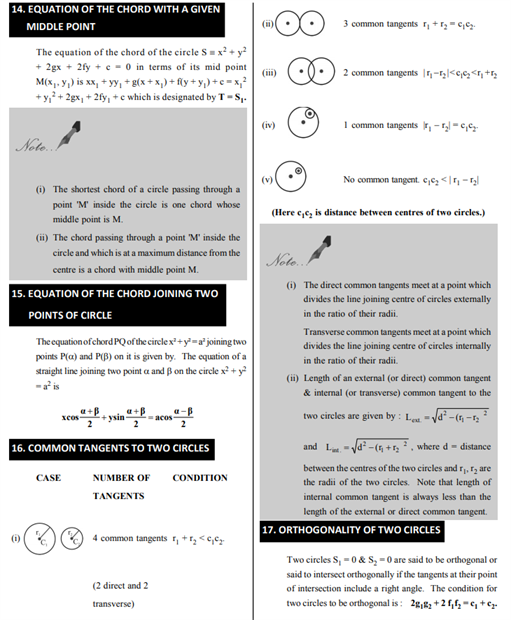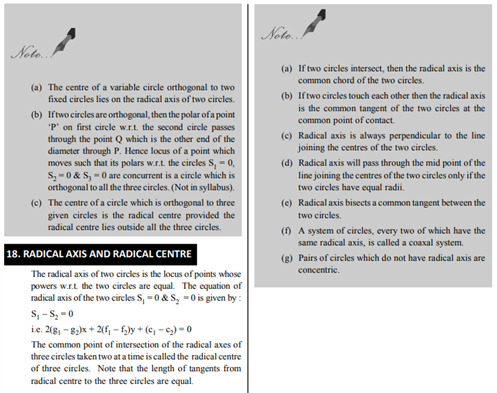# Revision Notes For CBSE Class 11 Maths Chapter 11 Conic Sections

Class 11 maths revision notes chapter 11 Conic Sections is very important if students are preparing for exams and need a reference material by their side. They will be made aware of all the curved 1-dimensional geometrical figures and their application along with formulas. We are aware of the equation of a circle that is:

• The center-radius form of the circle equation is in the format (x – h)2 + (y – k)2 = r2, with the center being at the point (h, k) and the radius is “r”. This form of the equation is helpful since you can easily find the center and the radius.

These equations if understood thoroughly with the help of these notes will prove to be helpful when it comes to solving practical and complex problems in later stages of academics. They will get to know about parabola, ellipse, hyperbola, circle along with their applications with examples.

You can get here the maths revision notes for class 11 chapter 11 Conic Sections here.